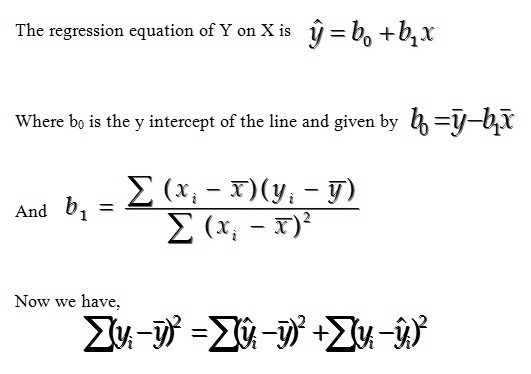# R Squared - Definition & Meaning

Published in Statistics by MBA Skool Team

## What is R Squared?

R Squared is popularly known as coefficient of determination and it gives an estimate of how well the data points will fit in a line or a curve. It may also be defined as the ratio of the variance due to explained reasons to the total variance.

It is of particular significance in statistical modeling and testing of hypothesis. Mathematically, say there is an independent variable X that assumes values xi and dependent variable Y that assumes values yi for every xi where i is from 1 to n.Where, Xbar is the mean of the independent variable and Ybar the mean of the dependent variable. The first term in the above equation is Total Sum of Squares (SST), the second is sum of squares due to regression (SSR) and the third is sum of squares due to error (SSE) such that

SST = SSR + SSE

The coefficient of determination (R squared) is the ratio of SSR to SST and hence explains the percentage of variance of the dependent variable that is due to the independent variable.

If we assume that gain in weight by children is dependent on the amount of food consumed and the coefficient of determination value is found to be 88%, then it shows strong regression relationship. We can say that 88% variability in the weight gained can be attributed to the linear relationship between weight gain & amount of food consumed.

Hence, this concludes the definition of R Squared along with its overview.

This article has been researched & authored by the Business Concepts Team. It has been reviewed & published by the MBA Skool Team. The content on MBA Skool has been created for educational & academic purpose only.

Browse the definition and meaning of more similar terms. The Management Dictionary covers over 1800 business concepts from 5 categories.